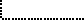Common Lisp the Language, 2nd EditionNext: Type Upgrading Up: Type Specifiers Previous: Type Conversion Function

# 4.9. Determining the Type of an Object

The following function may be used to obtain a type specifier describing the type of a given object.

[Function]
type-of object(type-of object) returns an implementation-dependent result: some type of which the object is a member. Implementors are encouraged to arrange for type-of to return the most specific type that can be conveniently computed and is likely to be useful to the user. If the argument is a user-defined named structure created by defstruct, then type-of will return the type name of that structure. Because the result is implementation-dependent, it is usually better to use type-of primarily for debugging purposes; however, in a few situations portable code requires the use of type-of, such as when the result is to be given to the coerce or map function. On the other hand, often the typep function or the typecase construct is more appropriate than type-of.Compatibility note: In MacLisp the function type-of is called typep, and anomalously so, for it is not a predicate.Many have observed (and rightly so) that this specification is totally wimpy and therefore nearly useless. X3J13 voted in June 1989 (TYPE-OF-UNDERCONSTRAINED)   to place the following constraints on type-of:

• Let x be an object such that (typep x type) is true and type is one of the following:

```array           float         package         sequence
bit-vector      function      pathname        short-float
character       hash-table    random-state    single-float
complex         integer       ratio           stream
condition       long-float    rational        string
cons            null          readtable       symbol
double-float    number        restart         vector
```

Then (subtypep (type-of x) type)) must return the values t and t; that is, type-of applied to x must return either type itself or a subtype of type that subtypep can recognize in that implementation.

• For any object x, (subtypep (type-of x) (class-of x)) must produce the values t and t.

• For every object x, (typep x (type-of x)) must be true. (This implies that type-of can never return nil, for no object is of type nil.)

• type-of never returns t and never uses a satisfies, and, or, not, or values type specifier in its result.

• For objects of CLOS metaclass structure-class or of standard-class, type-of returns the proper name of the class returned by class-of if it has a proper name, and otherwise returns the class itself. In particular, for any object created by a defstruct constructor function, where the defstruct had the name name and no :type option, type-of will return name.

As an example, (type-of "acetylcholinesterase") may return string or simple-string or (simple-string 20), but not array or simple-vector. As another example, it is permitted for (type-of 1729) to return integer or fixnum (if it is indeed a fixnum) or (signed-byte 16) or (integer 1729 1729) or (integer 1685 1750) or even (mod 1730), but not rational or number, because

```(typep (+ (expt 9 3) (expt 10 3)) 'integer)
```

is true, integer is in the list of types mentioned above, and

```(subtypep (type-of (+ (expt 1 3) (expt 12 3))) 'integer)
```

would be false if type-of were to return rational or number.Next: Type Upgrading Up: Type Specifiers Previous: Type Conversion Function

AI.Repository@cs.cmu.edu